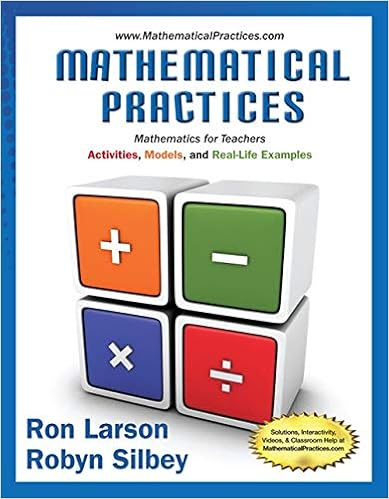# A quiz has 25 multiple choice questions each question

• Test Prep
• binghamton
• 8
• 50% (8) 4 out of 8 people found this document helpful

This preview shows page 4 - 8 out of 8 pages.

##### We have textbook solutions for you!
The document you are viewing contains questions related to this textbook.The document you are viewing contains questions related to this textbook.
Chapter 6 / Exercise 5
Mathematical Practices, Mathematics for Teachers: Activities, Models, and Real-Life Examples
LarsonExpert Verified
20. A quiz has 25 multiple choice questions. Each question has 5 possible answers, one of which iscorrect. A correct answer is worth 4 points, but a point is taken off for an incorrect answer. Astudent answers all questions by guessing at random. The students score is like the sum of 25draws from which one ofthe following boxes?(a)RightWrong(b)RightWrongWrongWrongWrong(c)4-1(d)40000(e)4-1-1-1-1
4
##### We have textbook solutions for you!
The document you are viewing contains questions related to this textbook.The document you are viewing contains questions related to this textbook.
Chapter 6 / Exercise 5
Mathematical Practices, Mathematics for Teachers: Activities, Models, and Real-Life Examples
LarsonExpert Verified
21. A box contains 2 red marbles and 3 blue ones. Five draws are made at randomwithoutreplace-ment. Which of the following is the chance that exactly 2 red marbles are drawn?
Part IVProblems22. (5 points) Find the number of ways of arranging 5 green balls and 4 yellow balls in a row. Simplifyyour answer to anumericalanswer, e.g.13.No credit will be given for not simplifying youranswer.23. (10 points) 400 draws with replacement are made from the following box:12345Approximate the chance of getting exactly 84 tickets with a 1 on it.5
24. A gambler decides to bet on the first 12 numbers on a roulette table. There are 12 chances in 38to win. This bet pays 2 to 1. The gambler bets \$3 on each play and plays 190 times.(a) (4 points) Construct a box model for one bet.(b) (2 points) Compute the expected value for the sum of all 190 plays.(c) (3 points) Compute the standard error for the sum of all 190 plays.(d) (5 points) What is the probability that the gambler leaves the roulette table after his 190plays with more money that when he arrived? (Hint: The sum of 190 plays>0)6
25. A large group of math 147 students get together to test probability theory. Each one rolls a die180 times, and counts the number of aces.(a) (4 points) Construct a box model for this problem. Include the number of draws.(b) (2 points) Find the expected value for the number of aces these students should get.(c) (4 points) Find the standard error for for the number of aces these students should get.(d) (5 points) About what percentage of those people should get counts in the range 22 to 35inclusive?7
26. A certain university has 30,000 students. Of these students 6,000 are math majors. As part of acampus survey, a simple random sample of400 students is chosen.(a) (2 points) Find the expected value for the percent of math majors in this sample.(b) (4 points) Find the standard error for the percent of math majors in this sample.(c) (5 points) Estimate the chance that between 17% and 23% of the sample will be math majors.8
•••